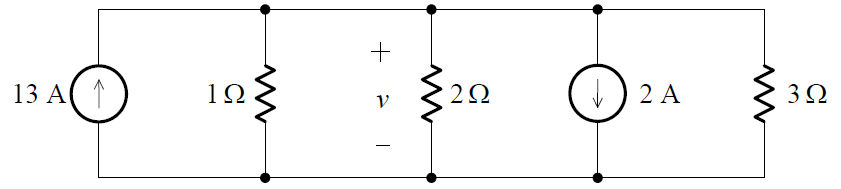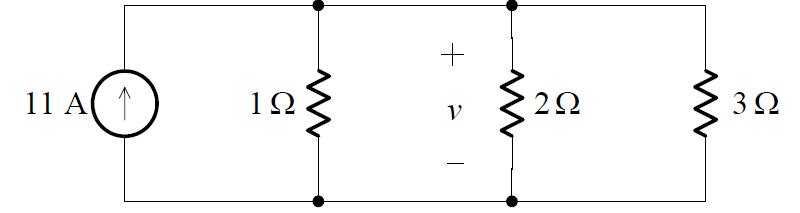# Combining Independent Current Sources in Parallel

It is not possible to combine independent current sources in series, since this would violate KCL. However, consider the parallel connection of two ideal current sources shown in (a) below:From KCL we find that i i1 + i2 , and by the definition of an ideal current source, this must always be the current into the arbitrary circuit. Thus, the parallel connection of two ideal current sources is equivalent to a single independent current source given by:Clearly, the obvious generalization to N current sources in parallel holds.

ExampleCombining the parallel independent current sources into a single equivalent source, we obtain the circuit:Since the equivalent resistance of the three resistors in parallel is given by:we obtain:

Req = 11/6 ohms

Then, from Ohm’s Law:

v =  (6/11) * 11 = 6 V

1.